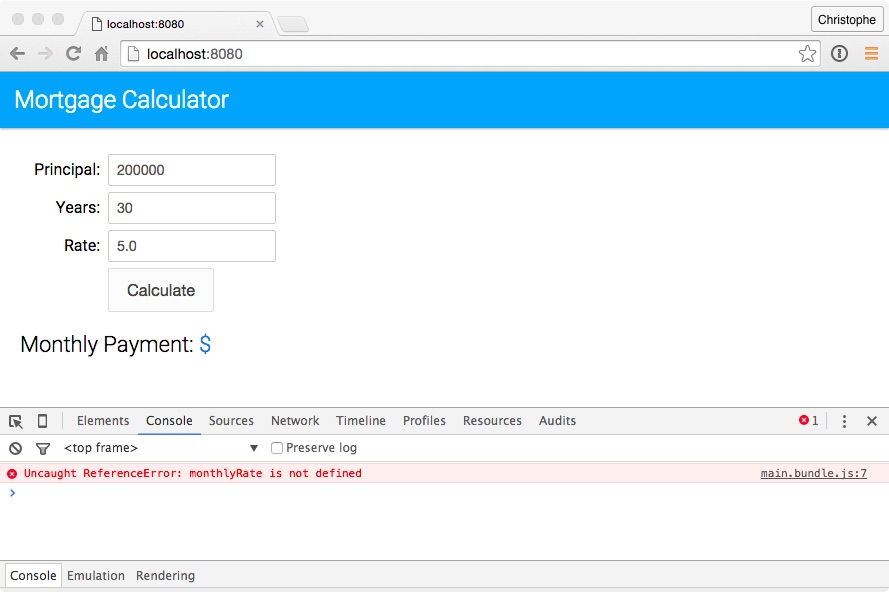# Using let Variables

ECMAScript 6 introduces a new keyword to declare variables: `let`. Unlike variables declared with `var` that are function-scoped, variables declared with `let` are block-scoped: they only exist in the block they are defined in.

In this unit, you modify the application to use `let` variables.

## Steps

1. In your code editor, open `js/main.js` and examine the `calculateMonthlyPayment` function:

``````var calculateMonthlyPayment = function(principal, years, rate) {
if (rate) {
var monthlyRate = rate / 100 / 12;
}
var monthlyPayment = principal * monthlyRate /
(1 - (Math.pow(1/(1 + monthlyRate), years * 12)));
return monthlyPayment;
};
``````

Notice that on line 5, the `monthlyRate` variable is available even though it was declared within the `if` block. This is because variables declared with `var` are function-scoped, and not block-scoped. This way of declaring and using variables is definitely not a best practice: it is used here to illustrate the difference between function-scoped and block-scoped variables.

2. Replace all the occurrences of `var` with `let`. Don’t change anything else yet.

3. On the command line, type the following command to run the babel script and compile `main.js` to ECMAScript 5:

``````npm run babel
``````
4. Open a browser, access http://localhost:8080, and click the Calculate button: it doesn’t work. Open the developer console. You should see a message similar to this:This is because unlike `var` variables which are function-scoped, `let` variables are block-scoped: they only exist in the block they are defined in.

5. In `main.js`, modify the `calculateMonthlyPayment` function as follows:

``````let calculateMonthlyPayment = function(principal, years, rate) {
let monthlyRate = 0;
if (rate) {
monthlyRate = rate / 100 / 12;
}
let monthlyPayment = principal * monthlyRate /
(1 - (Math.pow(1/(1 + monthlyRate), years * 12)));
return monthlyPayment;
};
``````
6. On the command line, type the following command to rebuild the application:

``````npm run babel
``````
7. Open a browser, access http://localhost:8080, and click the Calculate button: you should now see the monthly payment.